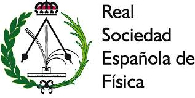#XXXVI Reunión Bienal de la Real Sociedad Española de Física

17-21 July 2017
Santiago de Compostela, Facultade de Química

## Parity violating electron scattering

17 Jul 2017, 18:55
20m
Aula Química Física (Facultade de Química (USC))

Nuclear Physics

### Speaker

Dr. Raul Gonzalez Jimenez (Ghent University)

### Description

Nucleons are not point like particles, they are made of quarks interacting each other by exchanging gluons. Gluons can be converted into quark-antiquark pairs giving raise to the sea quark. Quantum Chromodynamics (QCD) is the fundamental theory that describes the strong interaction; however, due to the complexity of the quark-gluon dynamics, QCD does not allow us to get analytical results in the energy region of interest to Nuclear Physics at low 4-momentum transfer, $Q^2$. Alternatively, the internal dynamics of the quarks inside the nucleon can be described in terms of several phenomenological functions (form factors) which depend on a single scalar variable: $Q^2$. The form factors are related to the electric, magnetic, axial and axial-vector charge distributions in the nucleon. The study and determination of these nucleon form factors can provide constraints to the microscopic models that aim to describe the nucleonic structure from QCD. Moreover, form factors are a main ingredient for the description of the lepton-nucleus/nucleon cross sections. One of the main objectives of our work is to improve the current knowledge on the structure of the nucleon through the study of the form factors. As tools, we have analyzed elastic electron-nucleon and quasielastic electron-nucleus scattering processes. These reactions provide information on the electromagnetic (EM) and weak neutral current (WNC) form factors. Although the EM interaction is dominant in electron-nucleon/nucleus scattering, i.e., parity conserving processes, the electron also feels the weak (neutral) interaction that does not conserve parity. Due to the strength of the weak interaction (several orders of magnitude lower than the electromagnetic one) to get information on the weak interaction is essential to analyze observables whose presence is due unequivocally to parity violating processes. Thus, we have studied the observable called parity violating asymmetry (${\cal A}^{PV}$) defined as ${\cal A}^{PV}=(\sigma^+ - \sigma^-)/(\sigma^+ + \sigma^-)$, where $\sigma^{+/-}$ represents the differential cross section (electron-proton or electron-nucleus) with positive/negative helicity of the incident electron. The PV asymmetry linked to the elastic electron-proton scattering (PVep asymmetry), $p(\vec{e},e')p$, is particularly sensitive to the electric and magnetic strange contributions. An statistical analysis of all PVep asymmetry data has also been presented. From this analysis we have got the highest precision constraint for the vector strange form factor compatible with the experimental data up to date. We have also presented a general study of the PV asymmetry associated to quasielastic electron-nucleus scattering processes (PVQE asymmetry). From our analyses we conclude that the PVQE asymmetry is an excellent observable to obtain nucleonic information on the axial-vector sector of the weak neutral current. Specifically, measurements of the PVQE asymmetry at backward scattering angles would provide information on the radiative corrections entering in the isovector axial-vector sector.

### Primary author

Dr. Raul Gonzalez Jimenez (Ghent University)

### Co-author

Prof. J.A. Caballero (Universidad de Sevilla)

### Presentation Materials

 Slides
###### Your browser is out of date!

Update your browser to view this website correctly. Update my browser now

×